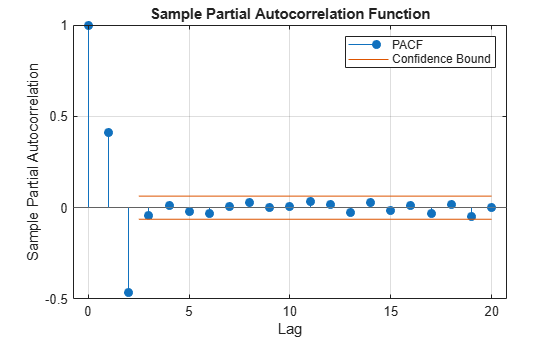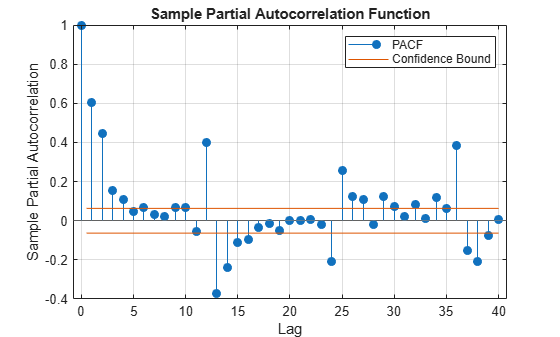# parcorr

Sample partial autocorrelation

## Syntax

``parcorr(y)``
``parcorr(y,Name,Value)``
``pacf = parcorr(___)``
``````[pacf,lags,bounds] = parcorr(___)``````
``parcorr(ax,___)``
``````[pacf,lags,bounds,h] = parcorr(___)``````

## Description

example

````parcorr(y)` plots the sample partial autocorrelation function (PACF) of the univariate, stochastic time series `y` with confidence bounds.```

example

````parcorr(y,Name,Value)` uses additional options specified by one or more name-value pair arguments. For example, `parcorr(y,'NumLags',10,'NumSTD',2)` plots the sample PACF of `y` for `10` lags and displays confidence bounds consisting of `2` standard errors.```

example

````pacf = parcorr(___)` returns the sample PACF of `y` using any of the input arguments in the previous syntaxes.```

example

``````[pacf,lags,bounds] = parcorr(___)``` additionally returns the lag numbers that MATLAB® uses to compute the PACF, and also returns the approximate upper and lower confidence bounds.```
````parcorr(ax,___)` plots on the axes specified by `ax` instead of the current axes (`gca`). `ax` can precede any of the input argument combinations in the previous syntaxes.```
``````[pacf,lags,bounds,h] = parcorr(___)``` plots the sample PACF of `y` and additionally returns handles to plotted graphics objects. Use elements of `h` to modify properties of the plot after you create it.```

## Examples

collapse all

Specify the AR(2) model:

`${y}_{t}=0.6{y}_{t-1}-0.5{y}_{t-2}+{\epsilon }_{t},$`

where ${\epsilon }_{t}$ is Gaussian with mean 0 and variance 1.

```rng(1); % For reproducibility Mdl = arima('AR',{0.6 -0.5},'Constant',0,'Variance',1)```
```Mdl = arima with properties: Description: "ARIMA(2,0,0) Model (Gaussian Distribution)" Distribution: Name = "Gaussian" P: 2 D: 0 Q: 0 Constant: 0 AR: {0.6 -0.5} at lags [1 2] SAR: {} MA: {} SMA: {} Seasonality: 0 Beta: [1×0] Variance: 1 ```

Simulate 1000 observations from `Mdl`.

`y = simulate(Mdl,1000);`

Compute the PACF.

```[partialACF,lags,bounds] = parcorr(y,'NumAR',2); bounds```
```bounds = 2×1 0.0632 -0.0632 ```

`bounds` displays (-0.0633, 0.0633), which are the upper and lower confidence bounds.

Plot the PACF.

`parcorr(y)`The PACF cuts off after the second lag. This behavior indicates an AR(2) process.

Specify the multiplicative seasonal ARMA $\left(2,0,1\right)×\left(3,0,0{\right)}_{12}$ model:

`$\left(1-0.75L-0.15{L}^{2}\right)\left(1-0.9{L}^{12}+0.75{L}^{24}-0.5{L}^{36}\right){y}_{t}=2+{\epsilon }_{t}-0.5{\epsilon }_{t-1},$`

where ${\epsilon }_{t}$ is Gaussian with mean 0 and variance 1.

```Mdl = arima('AR',{0.75,0.15},'SAR',{0.9,-0.75,0.5},... 'SARLags',[12,24,36],'MA',-0.5,'Constant',2,... 'Variance',1);```

Simulate data from `Mdl`.

```rng(1); y = simulate(Mdl,1000); ```

Plot the default partial autocorrelation function (PACF).

```figure parcorr(y)```The default correlogram does not display the dependence structure for higher lags.

Plot the PACF for 40 lags.

```figure parcorr(y,'NumLags',40)```The correlogram shows the larger correlations at lags 12, 24, and 36.

## Input Arguments

collapse all

Observed univariate time series for which the software computes or plots the PACF, specified as a vector. The last element of `y` contains the latest observation.

Specify missing observations using `NaN`. The `parcorr` function treats missing values as missing completely at random.

Data Types: `double`

Axes on which to plot, specified as an `Axes` object.

By default, `parcorr` plots to the current axes (`gca`).

### Name-Value Pair Arguments

Specify optional comma-separated pairs of `Name,Value` arguments. `Name` is the argument name and `Value` is the corresponding value. `Name` must appear inside quotes. You can specify several name and value pair arguments in any order as `Name1,Value1,...,NameN,ValueN`.

Example: `parcorr(y,'NumLags',10,'NumSTD',2)` plots the sample PACF of `y` for `10` lags and displays confidence bounds consisting of `2` standard errors.

Number of lags in the sample PACF, specified as the comma-separated pair consisting of `'NumLags'` and a positive integer. `parcorr` uses lags `0:NumLags` to estimate the PACF.

The default is ```min([20,T – 1])```, where `T` is the effective sample size of `y`.

Example: `parcorr(y,'Numlags',10)` plots the sample PACF of `y` for lags `0` through `10`.

Data Types: `double`

Number of lags in a theoretical AR model of `y`, specified as the comma-separated pair consisting of `'NumAR'` and a nonnegative integer less than `NumLags`.

`parcorr` uses `NumAR` to estimate confidence bounds. For lags > `NumAR`, `parcorr` assumes that `y` is a Gaussian white-noise process of length n. Consequently, the standard error is approximately $1/\sqrt{T},$ where T is the effective sample size of `y`.

Example: `parcorr(y,'NumAR',10)` specifies that `y` is an AR(`10`) process, and plots confidence bounds for all lags greater than `10`.

Data Types: `double`

Number of standard errors in the confidence bounds, specified as the comma-separated pair consisting of `'NumSTD'` and a nonnegative scalar. For all lags > `NumAR`, the confidence bounds are 0 ± `NumSTD*`$\stackrel{^}{\sigma }$, where $\stackrel{^}{\sigma }$ is the estimated standard error of the sample partial autocorrelation.

The default yields approximate 95% confidence bounds.

Example: `parcorr(y,'NumSTD',1.5)` plots the PACF of `y` with confidence bounds `1.5` standard errors away from 0.

Data Types: `double`

PACF estimation method, specified as the comma-separated pair consisting of `'Method'` and a value in this table.

ValueDescriptionRestrictions
`'ols'`Ordinary least squares (OLS)`y` must be a fully observed series (that is, it does not contain any `NaN` values)
`'yule-walker'`Yule-Walker equationsNone

If `y` is a fully observed series, then the default is `'ols'`. Otherwise, the default is `'yule-walker'`.

Example: `parcorr(y,'Method','yule-walker')` estimates the PACF of `y` using the Yule-Walker equations and then plots the PACF.

Data Types: `char` | `string`

## Output Arguments

collapse all

Sample PACF of the univariate time series `y`, returned as a numeric vector of length `NumLags` + `1`.

The elements of `pacf` correspond to lags 0,1,2,...,`NumLags` (that is, elements of `lags`). For all time series `y`, the lag 0 partial autocorrelation `pacf(1)` = `1`.

Lag numbers used for PACF estimation, returned as a numeric vector of length `NumLags` + `1`.

Approximate upper and lower partial autocorrelation confidence bounds assuming `y` is an AR(`NumAR`) process, returned as a two-element numeric vector.

Handles to plotted graphics objects, returned as a graphics array. `h` contains unique plot identifiers, which you can use to query or modify properties of the plot.

collapse all

### Partial Autocorrelation Function

The partial autocorrelation function measures the correlation between yt and yt + k after adjusting for the linear effects of yt + 1,...,yt + k – 1.

The estimation of the PACF involves solving the Yule-Walker equations with respect to the autocorrelations. However, if the time series is fully observed, then the PACF can be estimated by fitting successive autoregressive models of orders 1, 2,... using ordinary least squares. For details, see , Chapter 3.

### Missing Completely at Random

Observations of a random variable are missing completely at random if the tendency of an observation to be missing is independent of both the random variable and the tendency of all other observations to be missing.

## Tips

To plot the PACF without confidence bounds, set `'NumSTD',0`.

## Algorithms

`parcorr` plots the PACF when you do not request any output or when you request the fourth output.

 Box, G. E. P., G. M. Jenkins, and G. C. Reinsel. Time Series Analysis: Forecasting and Control. 3rd ed. Englewood Cliffs, NJ: Prentice Hall, 1994.

 Hamilton, J. D. Time Series Analysis. Princeton, NJ: Princeton University Press, 1994.